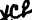Custom Search*C2 P A R    2 3 5 CHAPTER  6 REPAIR   AND   REBUILD   STANDARDS 2 3 5 .     G E N E R A L .   W e a r    L i m i t s    a n d    P o i n t s    o f    M e a s u r e- ment.   Data   covering   the   sizes   and   fits   of   new parts   and   wear   limits   information,   is   given   in pars.   236   through   249,   below.   Points   of   meas- u r e m e n t    o f    a l l    c r i t i c a l    d i m e n s i o n s    a r e    i n d i - c a t e d    b y    s m a l l    l e t t e r s    i n    t h e    e x p l o d e d    v i ew parts   groups,   figs.   373   through   386,   fold-outs 2   through   15. .   T - T i g h t ,    L - L o o s e .    T h e    " S i z e    a n d    F it of   New   Parts"   column   includes   the   manufacture dimensions   and   tolerances,   and   the   fits   of   mat- ing   parts.   T   denotes   a   tight   (interference)   fit; L   denotes   a   loose   (clearance)   fit. .   M e a n i n g    o f    W e a r    L i m i ts (1)   The   wear   limits   indicate   the   dimen- sions   to   which   a   part   may   wear   before   it   must be   replaced.   Normally,   any   part   not   worn   be- yond   its   wear   limit   will   be   approved   for   con- tinued   use,   if   it   is   not   otherwise   damaged. ( 2 )    A    s i n g l e    a s t e r i s k    ( * )    i n    t h e    w e a r limits   column   indicates   that   the   part   should   be replaced   when   worn   beyond   the   limits   stated in   "Size   and   Fit   of   New   Parts"   column. ( 3 )    A    d o u b l e    a s t e r i s k    ( * * )    i n d i c a t e s    a limit   applying   to   the   fit   between   mating   parts. In   such   cases,   wear   is   allowed   on   either   or both   mating   parts   so   long   as   the   fit   is   within the   specified   limit. .   S t a n d a r d     T o r q u e     L i s t i n g s .     S t a n d a rd torque   to   which   bolts,   nuts   and   screws   are   to be   tightened   is   listed   in   par.   250   under   Table V .     S T A N D A R D     T O R Q U E     S P E C I F I C A T I O N S. T h e s e    a r e    n o r m a l    t o r q u e    v a l u e s - s p e c i a l torques   are   listed   in   the   copy   pertinent   to   the a p p l i c a b l e    p a r t s . 2 0 2